# What are the reviews telling us?

This code have been lightly revised to make sure it works as of 2018-12-20.

In this post we will look at a handful of English1 movies reviews from imdb which I have scraped and placed in this repository movie reviews. I took a look at the best and worst rated movies with their best and worst reviews respectively. From that we will try to see if we are able to see how positive reviews on good movies are different then positive reviews on bad movies and so on.

We will use fairly standard packages with the inclusion of paletteer for the sole reason of self promotion. (yay!!!)

``````library(tidyverse)
## Warning: package 'tibble' was built under R version 3.6.2
library(tidytext)
library(plotly)
library(paletteer)``````

``reviews_raw <- read_csv("https://raw.githubusercontent.com/EmilHvitfeldt/movie-reviews/master/reviews_v1.csv")``

Lets take a look at the data I prepared for us:

``````glimpse(reviews_raw)
## Rows: 9,764
## Columns: 7
## \$ text          <chr> "It is a very boring and weird movie. Watch it only if …
## \$ id            <chr> "tt0012349", "tt0012349", "tt0012349", "tt0012349", "tt…
## \$ title         <chr> "The Kid", "The Kid", "The Kid", "The Kid", "The Kid", …
## \$ rating        <dbl> 8.3, 8.3, 8.3, 8.3, 8.3, 8.3, 8.3, 8.3, 8.3, 8.3, 8.3, …
## \$ url           <chr> "https://www.imdb.com/title/tt0012349/", "https://www.i…
## \$ movie_rating  <chr> "good", "good", "good", "good", "good", "good", "good",…``````

It include 7 different variables. There is some redundancy, the `url` variable contains the url of the movie, and `id` and `title` are just the extracts from the `url` variable. The `rating` variable is the average rating of the movie and will not be used in this analysis. Lastly we have the `review_rating` and `movie_rating` which will denote if the review is positive or negative and if the movie being reviewed is good or bad respectively.

Lets start by unnesting the words and get the counts. We also don’t want to look at all the stopwords and words that contains numbers, this it likely not a great number of words but we will exclude them for now anyways.

``````counted_words <- unnest_tokens(reviews_raw, word, text) %>%
count(word, movie_rating, review_rating) %>%
anti_join(stop_words, by = "word") %>%
filter(!str_detect(word, "\\d"))``````

And lets have a quick looks at the result:

``````counted_words %>% arrange(desc(n)) %>% head(n = 15)
## # A tibble: 15 x 4
##    word   movie_rating review_rating     n
##    <chr>  <chr>        <chr>         <int>
##  1 movie  bad          good           7504
##  3 movie  good         bad            5692
##  4 movie  good         good           5507
##  5 film   good         good           4701
##  6 film   good         bad            3926
##  8 film   bad          good           3023
## 10 time   good         good           1757
## 11 story  good         good           1496
## 12 people bad          good           1409
## 13 time   good         bad            1387
## 14 people good         bad            1292

And we notice that the word movie have been used quite a lot more in reviews of bad movies then in good movies.

## Log odds

We have a bunch of counts here and we would like to find a worthwhile transformation of them. Since we have the number of reviews for good movies and bad movies we would be able to find the percentage of words appearing in good movies. This would give us a number between 0 and 1, where the interesting words would be when the percentage is close to 0 and 1 as it would show that the word is being used more in one than another.

By doing this transformation to both the review scores and movie scores will give us the following plot:

``````counted_words %>%
mutate(rating = str_c(movie_rating, "_", review_rating)) %>%
select(-movie_rating, -review_rating) %>%
drop_na() %>%
ggplot() +
aes(movie_lo, review_lo) +
geom_text(aes(label = word))``````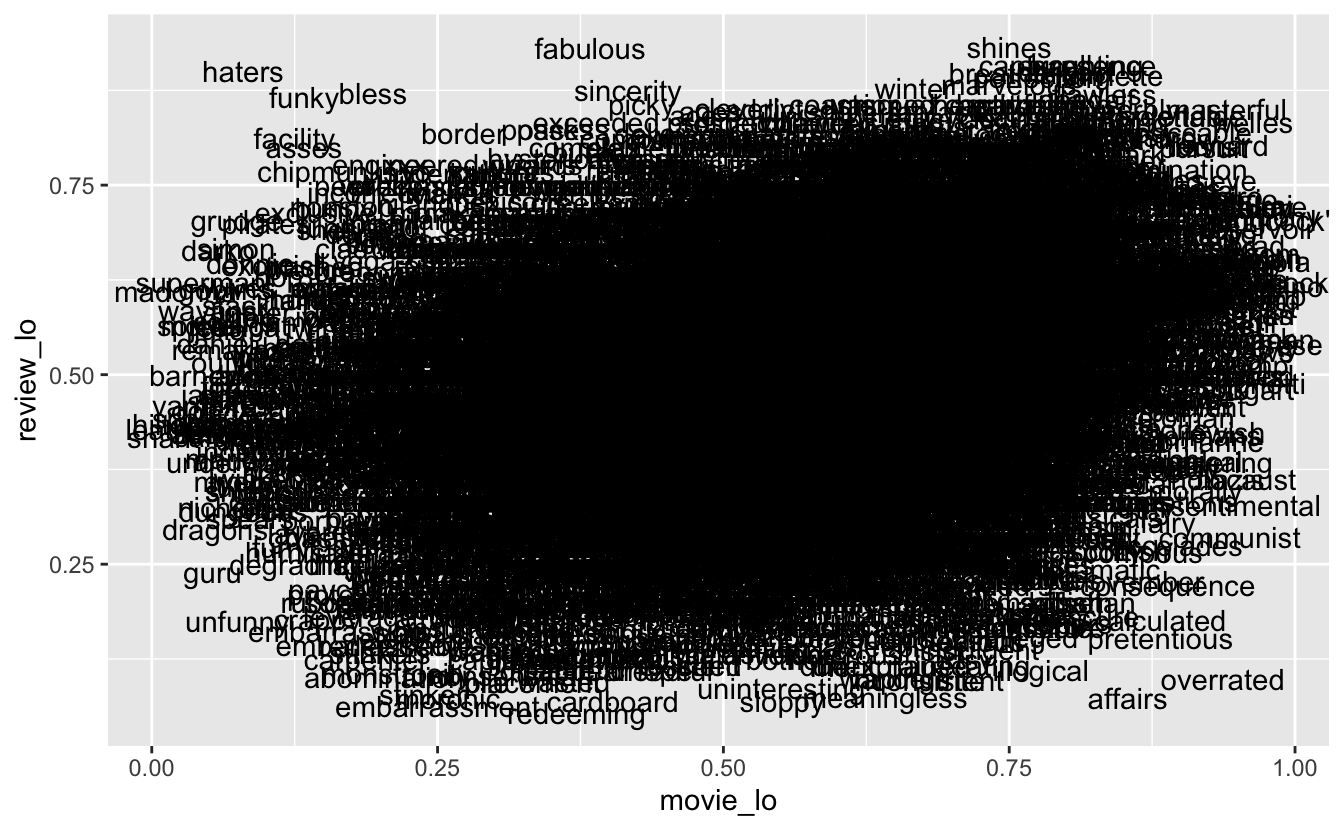Another way to do this is to take the log of the odds of one event happening over the other event. We will create this little helper function for us.

``````log_odds <- function(x, y) {
total <- x + y
p <- x / total
log(p / (1 - p))
}``````

applying this transformation instead expands the the limit from 0 to 1 to the whole number range where the midpoint is 0, this has some nice properties from a visualization perspective, it will also compact the center points a little more allowing outliers to be more prominent.

``````plot_data <- counted_words %>%
mutate(rating = str_c(movie_rating, "_", review_rating)) %>%
select(-movie_rating, -review_rating) %>%
drop_na() %>%
``````plot_data %>%
ggplot() +
aes(movie_lo, review_lo, label = word) +
geom_text()``````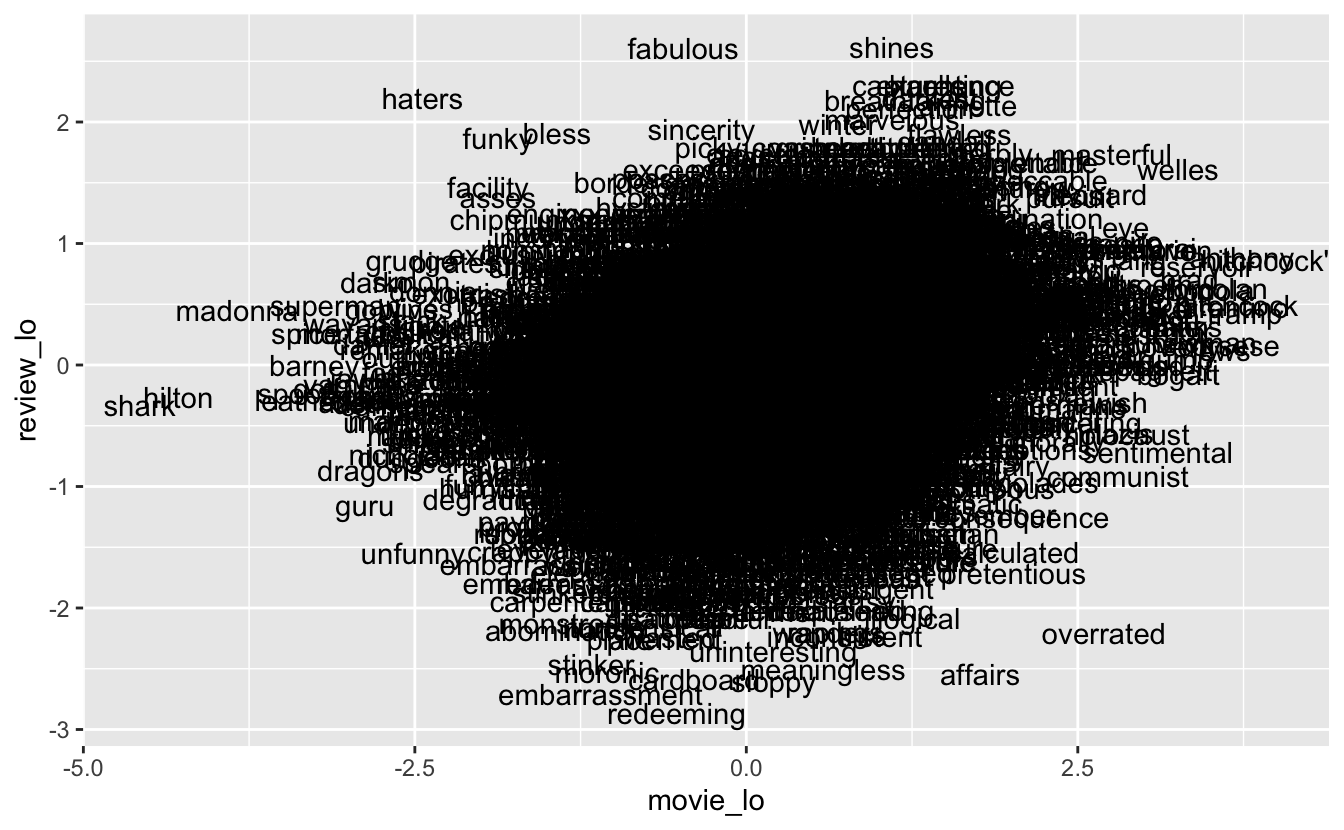We have a good degree of over plotting in this plot, but part of that might be because of the text, a quick look at the scatterplot still reveals a good deal of overplotting. We will try to counter that later on.

``````plot_data %>%
ggplot() +
aes(movie_lo, review_lo) +
geom_point(alpha = 0.5)``````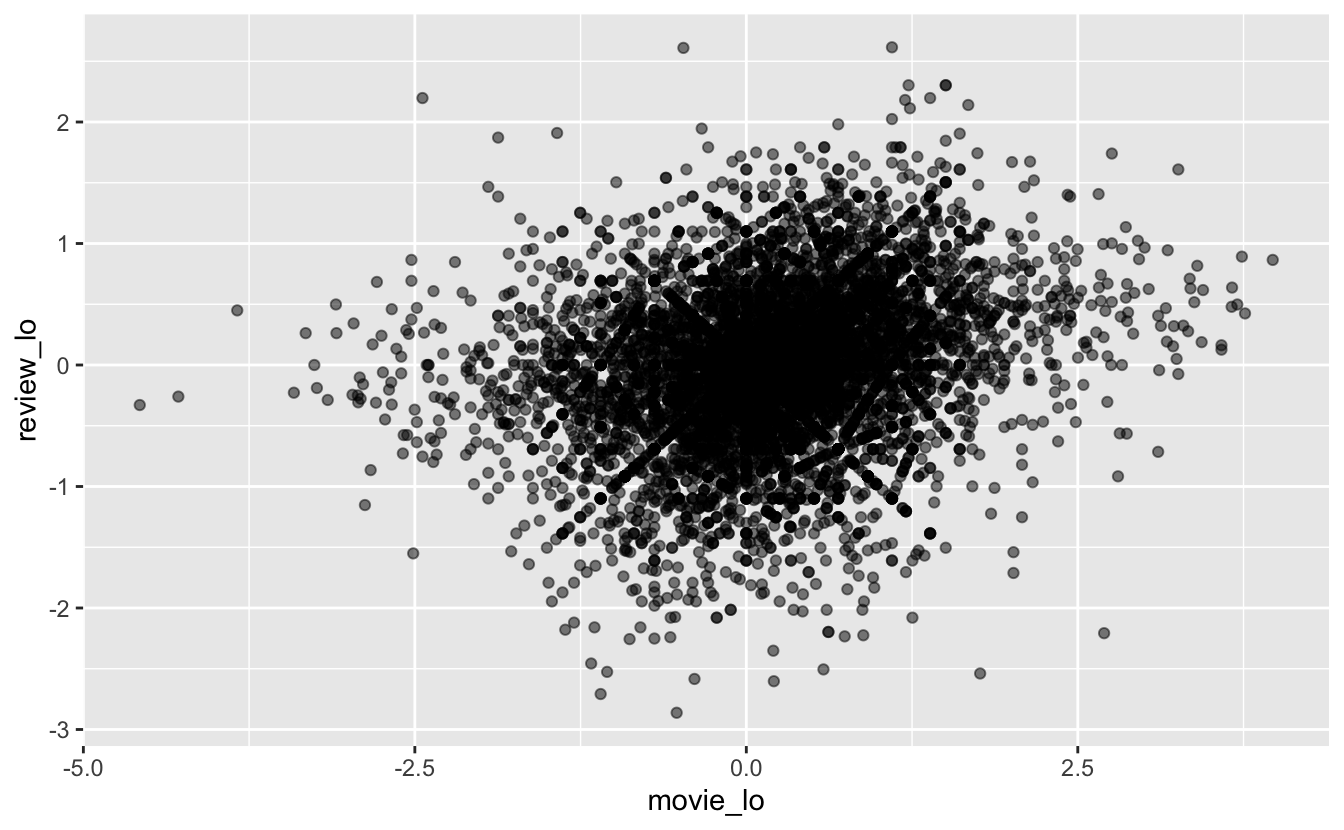Lets stay in the in the scatterplot. Lets tighten up the theme and include guidelines at y = 0 and x = 0. We will also find the range of the data to make sure we include all the points.

``````plot_data %>%
select(movie_lo, review_lo) %>%
range()
##  -4.574711  3.970292``````
``````plot_data %>%
ggplot() +
aes(movie_lo, review_lo) +
geom_vline(xintercept = 0, color = "grey") +
geom_hline(yintercept = 0, color = "grey") +
geom_point(alpha = 0.5) +
theme_minimal() +
coord_cartesian(ylim = c(-4.6, 4.6),
xlim = c(-4.6, 4.6)) +
labs(x = "← Bad Movies - Good Movies →", y = "← Bad Reviews - Good Reviews →")``````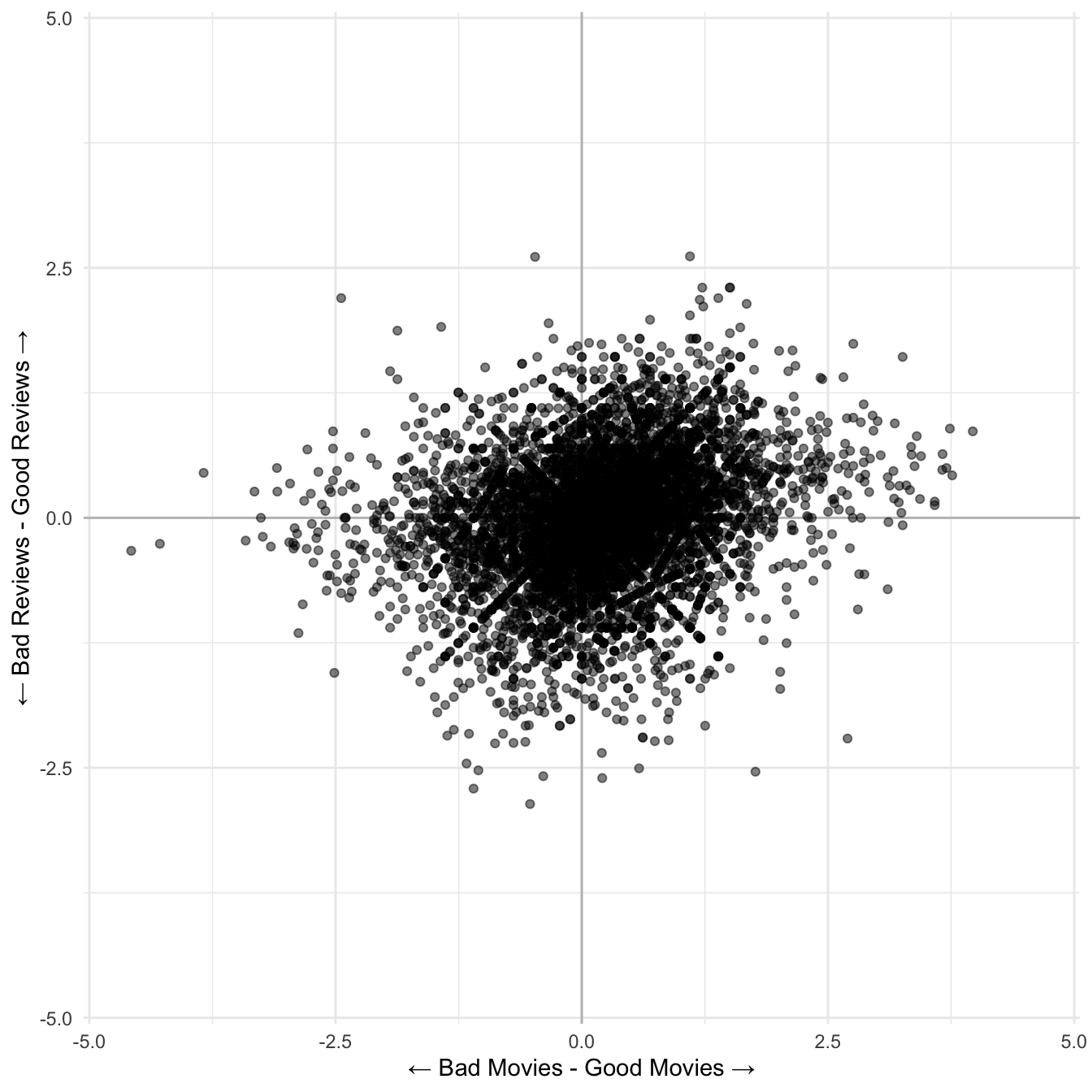We still have quite a bit of over plotting, I’m going to sample the points based on importance. The importance matrix I’m going to work with is the distance from the middle. In addition we are going to display the number of times a word is used by the size of the points.

``````set.seed(13)
plot_data_v2 <- plot_data %>%
mutate(distance = review_lo ^ 2 + movie_lo ^ 2,
sample_frac(0.1, weight = distance)

plot_data_v2 %>%
ggplot() +
aes(movie_lo, review_lo, size = n) +
geom_vline(xintercept = 0, color = "grey") +
geom_hline(yintercept = 0, color = "grey") +
geom_point(alpha = 0.5) +
theme_minimal() +
coord_cartesian(ylim = c(-4.6, 4.6),
xlim = c(-4.6, 4.6)) +
labs(x = "← Bad Movies - Good Movies →", y = "← Bad Reviews - Good Reviews →")``````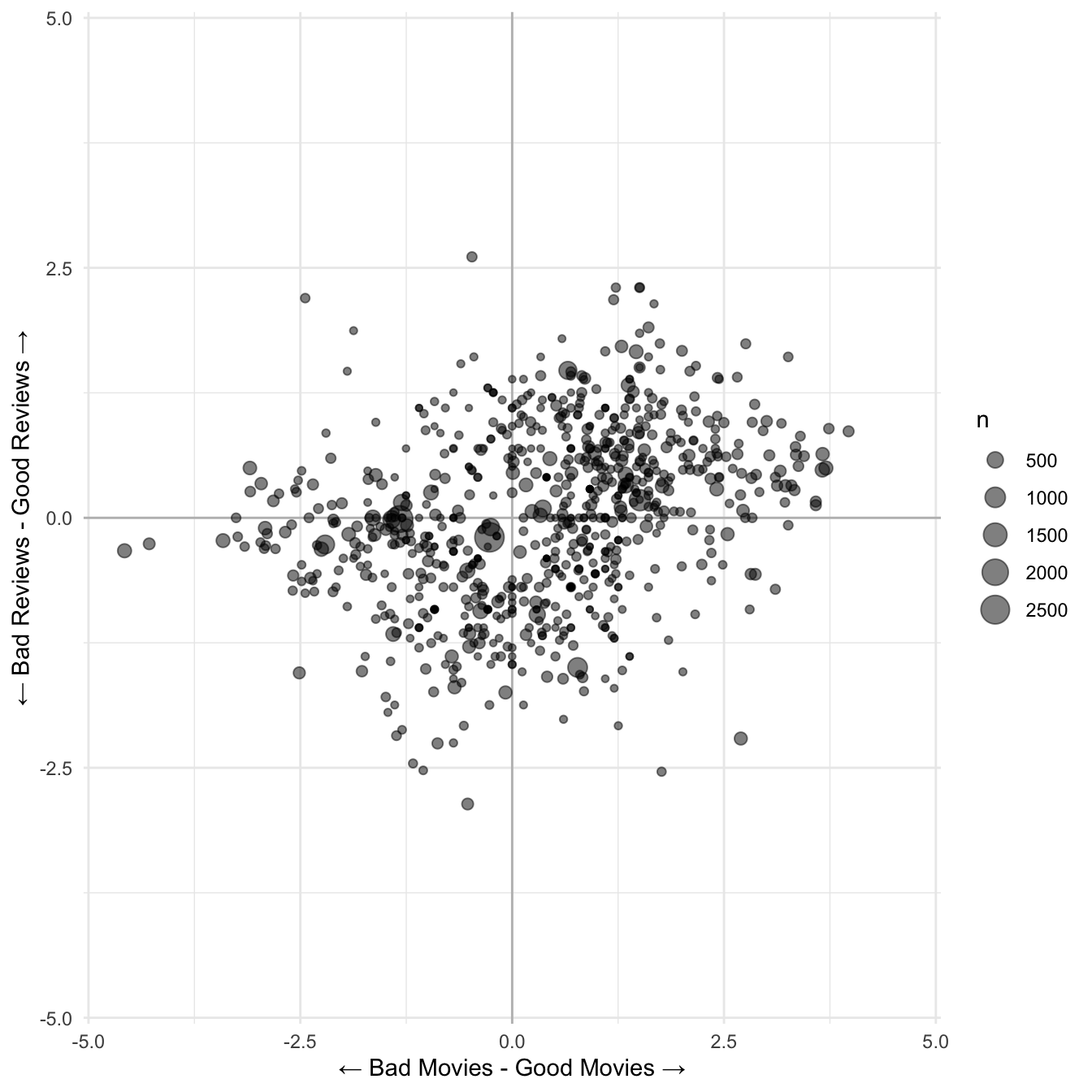Lastly we will make the whole thing interactive with plotly to allow hover text. We include some color to indicate distance to the center.

``````p <- plot_data_v2 %>%
ggplot() +
aes(movie_lo, review_lo, size = n, color = distance, text = word) +
geom_vline(xintercept = 0, color = "grey") +
geom_hline(yintercept = 0, color = "grey") +
geom_point(alpha = 0.5) +
theme_minimal() +
coord_cartesian(ylim = c(-4.6, 4.6),
xlim = c(-4.6, 4.6)) +
labs(x = "← Bad Movies - Good Movies →",
y = "← Bad Reviews - Good Reviews →",
title = "What are people saying about the best and worst movies on IMDB?") +
scale_color_paletteer_c("viridis::viridis") +
guides(color = "none", size = "none")

ggplotly(p, width = 700, height = 700, displayModeBar = FALSE,
tooltip = "text") %>%
config(displayModeBar = F)``````

And we are done and it looks amazing! With this dataviz we are able to see that the word overrated is mainly used in negative reviews about good movies. Likewise unfunny is used in bad reviews about bad movies. There is many more examples that I’ll let you explore by yourself.

Thanks for tagging along!